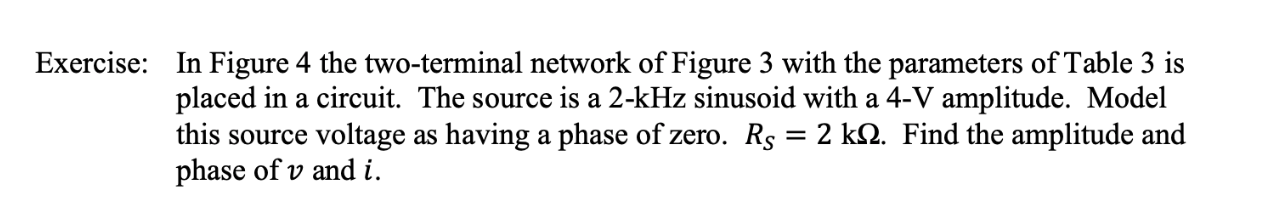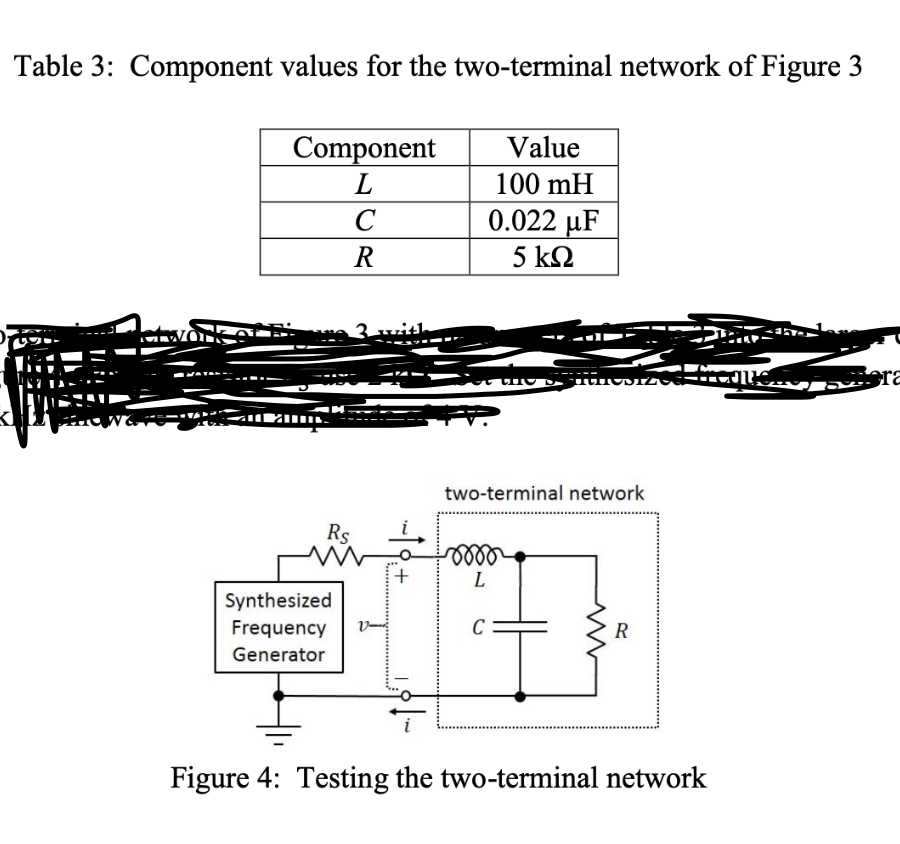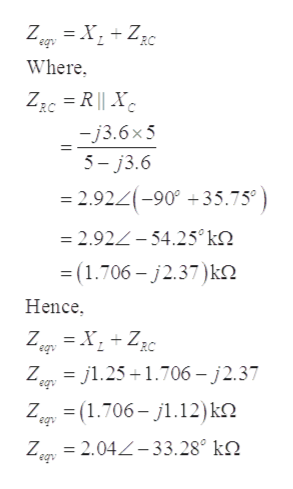# Exercise:In Figure 4 the two-terminal network of Figure 3 with the parameters of Table 3 isplaced in a circuit. The source is a 2-kHz sinusoid with a 4-V amplitude. Modelthis source voltage as having a phase of zero. Rs = 2 kQ. Find the amplitude andphase of v and i Table 3: Component values for the two-terminal network of Figure 3ValueComponentL100 mH0.022 uFC5 knN-two-terminal networkLSynthesizedCFrequencyv-RGeneratorFigure 4: Testing the two-terminal network-1

Question
4 viewshelp_outlineImage TranscriptioncloseExercise: In Figure 4 the two-terminal network of Figure 3 with the parameters of Table 3 is placed in a circuit. The source is a 2-kHz sinusoid with a 4-V amplitude. Model this source voltage as having a phase of zero. Rs = 2 kQ. Find the amplitude and phase of v and i fullscreenhelp_outlineImage TranscriptioncloseTable 3: Component values for the two-terminal network of Figure 3 Value Component L 100 mH 0.022 uF C 5 kn N- two-terminal network L Synthesized C Frequency v- R Generator Figure 4: Testing the two-terminal network -1 fullscreen
check_circle

Step 1

Redrawing the given circuit diagram, using the given points:

Step 2

Evaluating the parameters of the above diagrams using the given frequency of the 2-KHz and taking the sine wave voltage as the reference voltage.

Step 3

Referring the given circuit diagram, the resistance and the capacitance are in parallel to each other and t...help_outlineImage TranscriptioncloseZe=XZ2¢ eqy Where RC -j3.6x5 5-j3.6 = 2.924-90 +35.75 =2.924-54.25° k2 (1.706-j2.37)k Hence ZgrXZzc Zgrj.25 1.706-j2.37 Zig = (1.706-j1.12) k eqv eqv = 2.04Z-33.28° k2. Z fullscreen

### Want to see the full answer?

See Solution

#### Want to see this answer and more?

Solutions are written by subject experts who are available 24/7. Questions are typically answered within 1 hour.*

See Solution
*Response times may vary by subject and question.
Tagged in

### Electrical Engineering